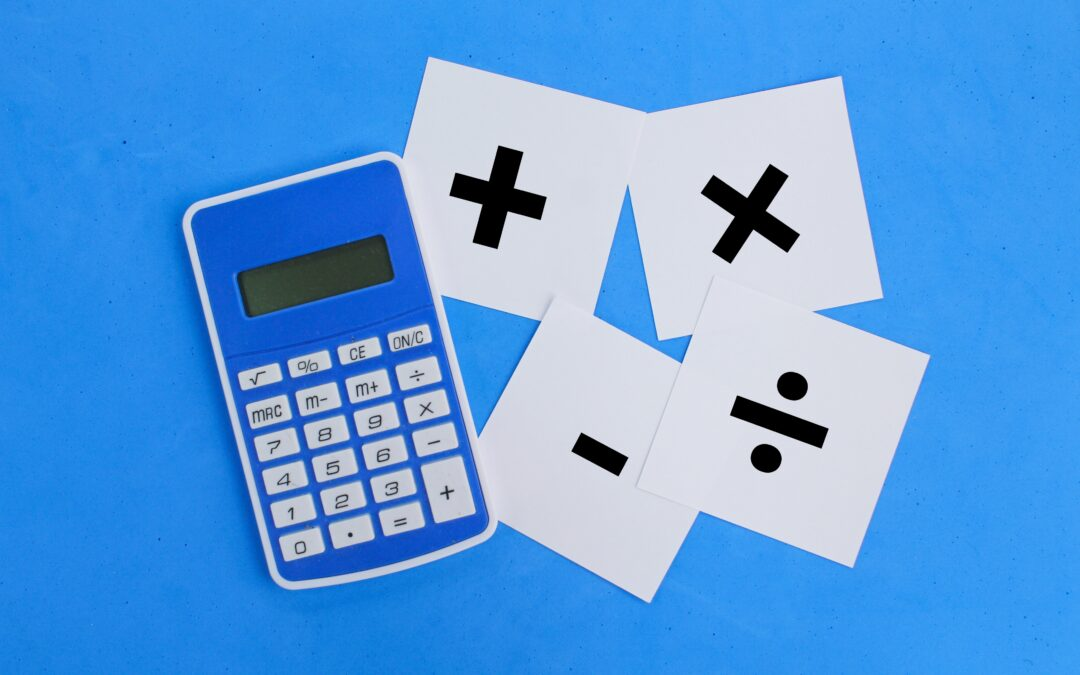Select Page## What Does Quotient Mean in Math?

Mathematics has a number of specified terminology that is used to describe certain types of numbers, equations, formulas, and more. So what does quotient mean in math? Well the quotient is the answer or result of dividing two numbers by each other. Lets look at some...## What Does Difference Mean in Math?

Mathematics can become quickly confusing as new concepts are introduced as students progress through higher and higher math courses. So what does difference mean in math? Well to put it shortly, the difference is the result of subtracting two or more separate numbers...